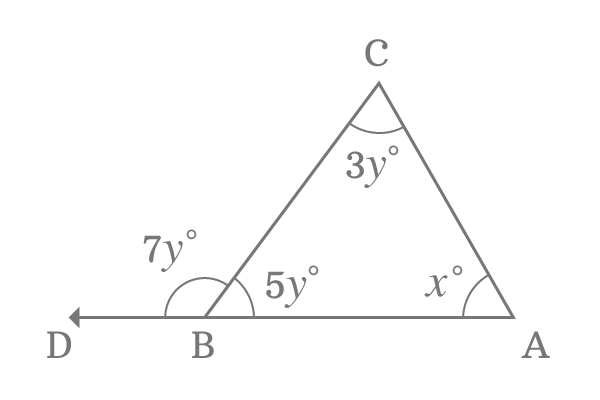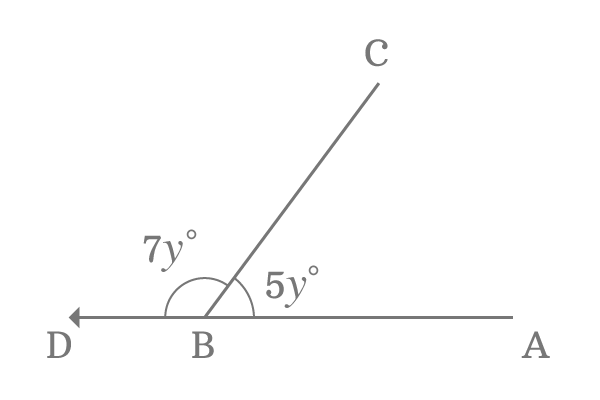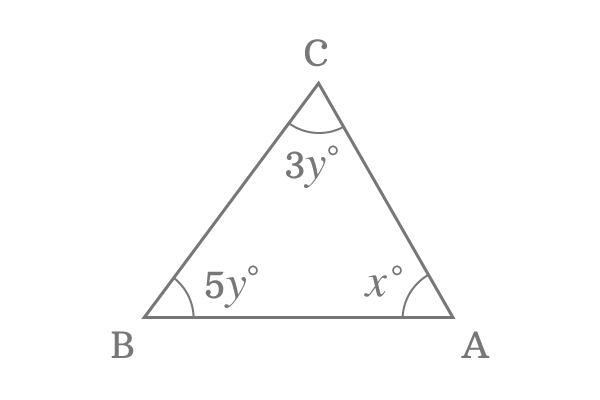# Find the value of x if interior angles are x°, 3y° and 5y° and exterior angle of a triangle is 7y°$\Delta ABC$ is triangle and its interior angles are $x^\circ$, $3y^\circ$ and $5y^\circ$. The side $\overrightarrow{AB}$ of the triangle is extended through a point $D$. Thus, the triangle $ABC$ has an exterior angle $CBD$ and it is $7y^\circ$.

In this geometry problem, the literals $x$ and $y$ are used to represent angles but they are symbolically written as $x^\circ$ and $y^\circ$. The meaning of representing $x$ and $y$ as $x^\circ$ and $y^\circ$ is the values of $x$ and $y$ are in degrees, which means the literal numbers $x$ and $y$ represent angles in degree measuring system.

Now, let us find the value of $x$ by using the geometrical relation between $y$ and $x$.

###### Step: 1

Forget about the triangle $ABC$ but only consider the intersecting lines $\overrightarrow{AD}$ and $\overline{BC}$. Geometrically, the line segment $\overline{BC}$ divides the angle $ABD$ into two parts and the angles are $5y$ and $7y$ in degrees.Therefore $\angle CBA = 5y$ and $\angle CBD = 7y$ in degrees.

The line $\overrightarrow{AD}$ is a straight ray. So, the angle of the line $\overrightarrow{AD}$ is $\angle ABD$ and it is a straight angle. Therefore, the angle $ABD$ is $180^\circ$ geometrically.

Geometrically, the sum of the angles $\angle CBA$ and $\angle CBD$ is equal to $\angle ABD$.

$\angle CBD + \angle CBA = \angle ABD$

$\implies 7y + 5y = 180^\circ$

$\implies 12y = 180^\circ$

$\implies y = \dfrac{180^\circ}{12}$

$\implies \require{cancel} y = \dfrac{\cancel{180^\circ}}{\cancel{12}}$

$\therefore \,\,\,\,\,\, y = 15^\circ$

Therefore, the value of $y$ is $15^\circ$.

###### Step: 2

The value of literal $y$ in degrees is calculated in previous step and it is $15^\circ$. Now, consider only $\Delta ABC$. Geometrically, it is proved that the sum of three angles of a triangle is $180^\circ$.It can be expressed in mathematical form as follows.

$\angle CBA + \angle BAC + \angle ACB = 180^\circ$

Substitute the values of all three angles of triangle $ABC$ in algebraic form.

$\implies 5y + x + 3y = 180^\circ$

$\implies x + 5y + 3y = 180^\circ$

$\implies x + 8y = 180^\circ$

$\implies x = 180^\circ -8y$

$\implies x = 180^\circ -8 (15^\circ)$

$\implies x = 180^\circ -120^\circ$

$\therefore \,\,\,\,\,\, x = 60^\circ$

Therefore, the value of $x$ is $60^\circ$ and it is the required solution for this geometric problem.

Latest Math Topics
Latest Math Problems

A best free mathematics education website for students, teachers and researchers.

###### Maths Topics

Learn each topic of the mathematics easily with understandable proofs and visual animation graphics.

###### Maths Problems

Learn how to solve the maths problems in different methods with understandable steps.

Learn solutions

###### Subscribe us

You can get the latest updates from us by following to our official page of Math Doubts in one of your favourite social media sites.